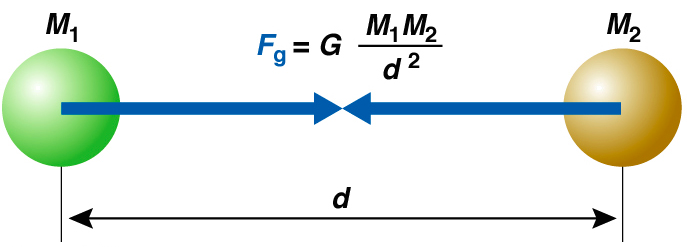# Force

Force:

Force is defined as a push or pulls that change or tends to the state of the body whether it is at rest or in uniformmotion along the straight line. Force is a vector quantity.

Newton law of gravitational: Newton’s Law of Gravitation states that “The force of attraction between the two bodies is directly proportional to the product of their masses and inversely proportional to the square of the distance between their centers.”Newton’s law of the gravitation holds true or applicable for all the objects present in this universe whether the objects be terrestrial or celestial. The gravitational force exists everywhere in this universe. Therefore Newton’s law of gravitation is called universe law.Verification of Newton’s law:

Consider the two bodies of the mass Mand M2with force F acting between them towards their centre.  If the distance between their centers is d then,

According to Newton’s universal law of gravitation,

We have F ∝  M1M2……1

F ∝   1/d2

Combining I and 2 we get F  ∝   M1 M2/d2

F=GM1M2/d2

Where G is proportionality constant which is known as universal gravitational constant.

The numerical value of gravitational constant (G) is 6.67*10-11 and its unit is Nm2/Kg2

Properties of G:

Value of G is independent of the temperature, pressure, nature of the intervening medium and chemical composition of the masses of the bodies.

Gravity:

It is the force which pulls an object towards the centre of the earth.

Acceleration due to gravity: The acceleration produced in a freely falling body due to the influence of gravity is called Acceleration due to gravity.

Its symbol is “g” and its SI unit is meter per second (m/s2).

Relation between acceleration due to gravity and radius of the earth

Let M be the mass and R be the radius of the earth and m be the mass of the body kept at the surface of the earthAccording to the Newton’s law of gravitation the force of attraction between them is given by

F=G Mm/R2………………………1

Also the body is attracted towards the centre of the earth with a force given by

F=mg…………………..2

From 1 and 2, mg = G Mm /R2

g=GM/R2…………….3

G and M are constant whereas R varies because radius of the earth is more at the equator than at poles.

From 3

We can say that the acceleration due to the gravity is independent to the mass of the body but depends on the mass and radius of the earth.

Variation of the value of g:

a. Variation due to the shape of the earth: g ∝ 1/R2

Value of the g maximum at poles but minimum at equator

b.Variation due to the height from the surface of the earth: g’ = (R/R+h) 2 g

If we increase the height from the surface of the earth the quantity in the bracket becomes less than 1.

And the acceleration due to the gravity decreases as height from the surface is increased.

c. Variation from the depth of earth surface: g’ = g(R-x)/R since the quantity

(R-x)/R is less than 1 .The value of g insidethe earth decreases with increase in depth.

At centre of the earth x=R so gravity at the centre of the earth is 0

Gravitational field and Gravitational field intensity:

The space around the mass where gravitational force of influence of the mass can be felt is called gravitational field.

Gravitational field intensity is the force experienced by the unit mass kept at that point.

It is given by

Gravitational Field intensity (I) = Forced experienced / mass

If the body of mass m is kept at the distance d from the centre of the earth of mass M and radius R

Figure:

Them force experienced by the mass is given by

F = G Mm/d2

To calculate the gravitational field intensity we have to take a unit mass so m =1

Gravitational field intensity (I) = GM/d2

If the body is at the surface of the earth d = R

(I) = G M/R2

This is numerically equal to the acceleration due to gravity.

Mass: It is the quantity of matter contained in the body. It is scalar quantity.

Weight: It is the force with which the object is attracted towards the centre of the earth. It is vector quantity.

Weight=mass * gravity. Weight depends upon gravity and gravity depends on the distance.

E.g. As the stone is away from the earth surface the weight of the stone is less than the stone present in the bottom.

Free fall: When an object is falling towards the surface of the earth only under the influence of gravity without external resistance, the fall of the object is free fall.

Weightlessness: Weightlessness is the condition at which the apparent weight of a body is zero. Weightlessness is possible in the absence of gravity.